Courses

# Test: Visualising Solid Shapes - 3

## 20 Questions MCQ Test Mathematics (Maths) Class 7 | Test: Visualising Solid Shapes - 3

Description
This mock test of Test: Visualising Solid Shapes - 3 for Class 7 helps you for every Class 7 entrance exam. This contains 20 Multiple Choice Questions for Class 7 Test: Visualising Solid Shapes - 3 (mcq) to study with solutions a complete question bank. The solved questions answers in this Test: Visualising Solid Shapes - 3 quiz give you a good mix of easy questions and tough questions. Class 7 students definitely take this Test: Visualising Solid Shapes - 3 exercise for a better result in the exam. You can find other Test: Visualising Solid Shapes - 3 extra questions, long questions & short questions for Class 7 on EduRev as well by searching above.
QUESTION: 1

### How many edges does the following figure have?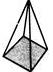Solution:

8 edges -4 sides of square and 4 sides of triangular faces,

QUESTION: 2

### Which of the following figures has six faces?

Solution:

The number effaces of a cube is 6.

QUESTION: 3

### Which shape is divided into two EQUAL parts by the dotted line?

Solution:

Observe the given figures. In the figure in option [d], the shape is divided equally.

QUESTION: 4

How many triangles can be seen in this figure?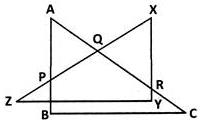Solution:The five triangles are, APQ, QXR, PZB, ABC and XYZ.

QUESTION: 5

Which of the following pair of shapes, when joined together (by placing them edge to edge) can form a rectangle?

Solution:

Clearly, the two halves of rectangles when  joined edge to edge form a rectangle.

QUESTION: 6

Which of the following is different from the other three?

Solution:

This figure has 5 lines unlike all other figures which have four sides.

QUESTION: 7

A solid object when seen from one side, looks like this.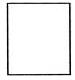The same solid, when viewed from top, looks like this.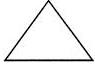Which of these shapes could it be?

Solution:
QUESTION: 8

How many corners does the shape given have?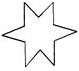Solution:
QUESTION: 9

Identify the correct statement from the following.

Solution:
QUESTION: 10

Rakesh has 10 one rupee coins of similar kind. He puts them exactly one on the other. What shape will he get finally?

Solution:

10 one-rupee coins piled up results in a cylinder.

QUESTION: 11

Identify the false statement from the following.

Solution:
QUESTION: 12

Match the following.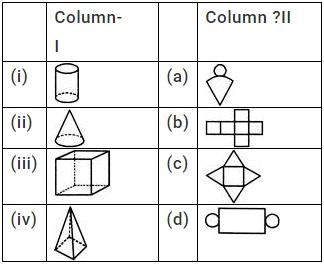Solution:

(i)Since cylinder is made up of two circles and a rectangle it matches with (d)
(ii)Since cone is made up of one circle and a triangle it matches with (a)
(iii)Since cuboid is made up of 6 rectangles,it matches with (b)
(iv) Since square pyramid is made up of 4 triangles and a square it matches with (c)

QUESTION: 13

Which of the following is an oblique sketch of a cube of edge 4 cm?

Solution:

A cube of edge 4 cm has square faces of side 4 cm.

QUESTION: 14

What is the total on the face opposite to  4+3 on the dice given?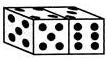Solution:

The sum of numbers on opposite faces is 7. So, the total on the face opposite to 4+3  is 3+4=7

.

QUESTION: 15

What is the number on the face opposite to 5 on a die?

Solution:

The number on opposite faces of a die add up to 7. So, the number on the face opposite to 5 is 2.

QUESTION: 16

The oblique sketch of 3 dice each with 2 cm edge placed side by side is given. What are its respective length, breadth and height?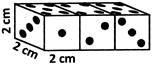Solution:

The height and width of the cuboid formed by placing 3 dice remain the same. Only length will be tripled.

QUESTION: 17

A die is cut horizontally. What is the cross-section obtained?

Solution:

The cross section of a cube is a square.

QUESTION: 18

The following figure shows a source of light (L), a solid (S) and its shadow (P) on a screen (M).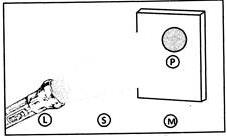What is the shape of P?

Solution:

The 2-D image of a sphere (ball) is a circle.

QUESTION: 19

The shadow of a 3-D object is given.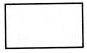Which of following could the object be?

Solution:

A book is cuboidal whose shadow is a rectangle.

QUESTION: 20

The following is the shadow of a 3-D object.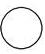Which of these objects does the shadow belong to?

Solution:

The pipe is cylindrical whose shadow is circular (front view).